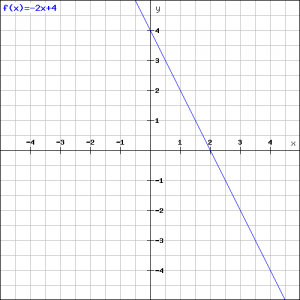# Solution assignment 02 Linear functions and graphs

### Assignment 2

Draw the line: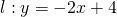and calculate the slope and the exact intersection point of the line with the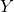-axis.

### Solution

The slope is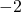(de coefficient van).
The easiest way to draw the line is finding two points on the line. The line through these two points is the one.
For the first point on the line we take the point with coordinates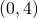, since the line intersects the-axis in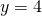.
You can find the second point by substituting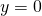in the formula. Then the following equation has to be solved: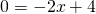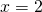and thus the intersection point with the-as has the coordinates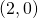. Now the line can be drawn easily.